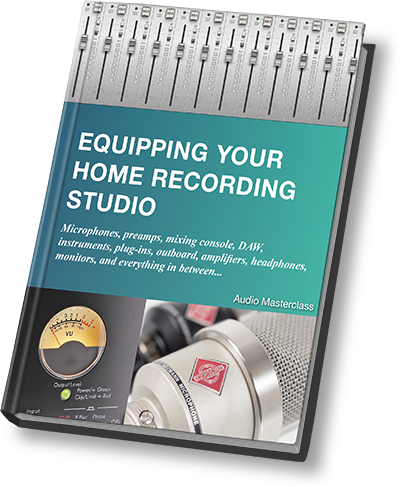## If you thought decibels were difficult, then think again. Sound engineers use the word decibel a hundred times a day. Don't you think you ought to know what it means?There's a formula for decibels...

dB = 20log10(V1/V2)

Too complicated? No matter...

Decibels can compare acoustic sound pressure levels, electrical voltages, magnetic levels on tape, the level of an optical film soundtrack or the movements in a record player stylus under the influence of the groove. So they are very versatile and worth understanding.

## FREE EBOOK - Equipping Your Home Recording StudioIf you can't get your head around the formula, then there are some easy values to remember...

• 0 dB = same level or no change in level
• +6 dB = 2x
• +12 dB = 4x
• +18 dB = 8x
• +20 dB = 10x
• +26 dB = 20x
• +40 dB = 100x
• +60 dB = 1000x
• +80 dB = 10,000x

Each time you add six decibels the voltage of the signal is multiplied by two. Each time you add twenty decibels the voltage is multiplied by ten. Decibels add, voltages (and all the other ways you can measure a signal) multiply.

Maybe you can see a pattern. If I told you that 66 dB is the same as multiplying the voltage of a signal by 2000, and you can see why, then you understand what's happening here.

Decibels can be negative too...

• 0 dB = same level or no change in level
• -6 dB = /2 (divide by 2)
• -12 dB = /4
• -18 dB = /8
• -20 dB = x 0.1
• -40 dB = x 0.01
• et cetera

So if the voltage coming out of a microphone is 10 millivolts (ten one-thousandths of a volt) and the gain of the preamp is set to 40 dB, then the output from the preamp is 1 nice round volt.

Easy peasy.